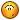BrainDen.com - Brain Teasers
• 0## QuestionThis may have been posted before.

How high can you count using four fours. You can use all mathematical symbols etc. and concatenate the 4's e.g. 4444. here's a start,

44/44 =1

4/4 + 4/4 =2

(4+4+4)/4 =3

There is a record for the highest number achieved somewhere, I'm sure one of you will know where.

• Created

## Recommended Posts

• 04 + (4-4)*4 = 4

##### Share on other sites
• 0(4*4 + 4)/4 = 5

(4 + 4)/4 + 4 = 6

44/4 - 4 = 7

4 + 4 + 4 - 4 = 8

4 + 4 + 4/4 = 9

(44 - 4)/4 = 10

##### Share on other sites
• 04(4-4) + 4

##### Share on other sites
• 0ceiling(4* 4√44) = 11 ?? Can we use ceiling?

##### Share on other sites
• 044 / (sqrt(4*4)) = 11

##### Share on other sites
• 044 / (sqrt(4*4)) = 11

Similar to another post this past week, is 'sqrt' inherently using a 2?

##### Share on other sites
• 0(44 + 4)/4 = 12

4! – 44/4 = 13

(4!)/4 + 4 + 4 = 14

4*4 – 4/4 = 15

4*4 + 4 – 4 = 16

4*4 + 4/4 = 17

##### Share on other sites
• 0(4! + 4)/4 + 4 = 11

##### Share on other sites
• 0(4P4) - 4!/4 = 18 if probability is allowed

4! – 4 – 4/4 = 19

4! – 4 + 4 – 4 = 20

4! – 4 + 4/4 = 21

4! – (4 + 4)/4 = 22

4! – 4^(4 – 4) = 23

4! + (4 – 4)*4 = 24

4! + 4^(4 – 4) = 25

4! + (4 + 4)/4 = 26

4! + 4 – 4/4 = 27

4! + 4 – 4 + 4 = 28

4! + 4 + 4/4 = 29

##### Share on other sites
• 0Infinite (No limit) - (4*Sigma(1 to x)of 1)/4 - just put what x you want and expand it it will give that number##### Share on other sites
• 04! + (4*4(4+4))/4 = 30

44/4 + 4! - 4 = 31

(4^4)/(4+4) = 32

(4/4 + 4^4)/(4+4) = 33

(4^4 * 4^4)/(4+4) = 34

(4^4)/(4+4) + ((4+4+4)/4) = 35

##### Share on other sites
• 04! + (4*4(4+4))/4 = 30

44/4 + 4! - 4 = 31

(4^4)/(4+4) = 32

(4/4 + 4^4)/(4+4) = 33

(4^4 * 4^4)/(4+4) = 34

(4^4)/(4+4) + ((4+4+4)/4) = 35

four fours?

##### Share on other sites
• 04 x (4+4) + 4 = 36

44 - 4- 4= 36

Edited by Andrew1258
##### Share on other sites
• 04! + ((4!+sqrt 4)/sqrt4) = 37

Edited by Forcedhand
##### Share on other sites
• 04444!

if someone can come up with something bigger i'd like to see it.

##### Share on other sites
• 0(4^4)^(4^4) = 256^256 = more than my calculator can handle

Ohhh... oops. You mean actually count.Edited by Dutch Riddle Amateur
##### Share on other sites
• 04444!

if someone can come up with something bigger i'd like to see it.

The title is "How high can you count" if you you are going to propose 4444! then please show how you calculated all the numbers below that!

##### Share on other sites
• 04!-(4-4/4)!=18 4!-(4+4/4)!=30

##### Share on other sites
• 0Well since the OP hasn't discredited sqrt or permutations/combinations, I can get up to 30. I'm stuck on 31.

nobody - could you check your 30?

Edited by ljb
##### Share on other sites
• 04! + 4 + 4 - sqrt 4

##### Share on other sites
• 0((4+sqrt 4)!+4!)/4!

##### Share on other sites
• 04^4/(4+4)

##### Share on other sites
• 0I doubt this valid

((4+4)*4) + sqrt(sqrt(sqrt(... to infinity...sqrt(4))))...))))

##### Share on other sites
• 0arcsin of 1/2 yields pi/6, not 30.

((4-4/4)!)!/4!

Edited by DarthNoob

## Join the conversation

You can post now and register later. If you have an account, sign in now to post with your account.×   Pasted as rich text.   Paste as plain text instead

Only 75 emoji are allowed.

×   Your previous content has been restored.   Clear editor

×   You cannot paste images directly. Upload or insert images from URL.

×